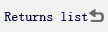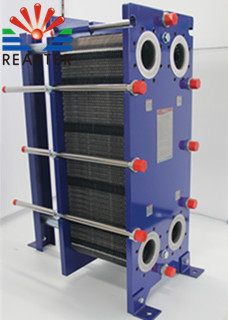﻿ The temperature difference design of the heat exchanger

#Location: Home >Latest news

# The temperature difference design of the heat exchangerReffer：ReapterClick number：- Release time：2021-04-01

What are the methods for heat exchanger temperature difference design? The heat exchanger manufacturer introduces us to this knowledge:1. Calculate the necessary heat exchange area. Go back to calculate the heat transfer area and check the pressure drop. The allowable pressure drop is often a limiting factor in the design of the heat exchanger. High-efficiency design always requires sufficient allowable pressure drop. If the relationship between heat transfer load, flow rate and allowable pressure drop cannot be coordinated no matter how the process layout is changed, only inefficient design schemes can be made.

2. Select the heat exchanger process and the number of channels, initially select the cold and hot fluid process and the number of channels, and calculate the flow rate from this.

3. Estimate the heat exchange area, and calculate the approximate heat exchange area based on the heat exchange amount.

4. Choose the appropriate plate model, and select the appropriate plate model according to the heat exchange and flow rate. The choice of plate model depends on the specific working conditions. When the flow rate is large and the pressure drop is low, the plate type with lower resistance should be selected; otherwise, the plate type with a slightly larger resistance coefficient can be used.

5. Finding the average temperature difference of the heat exchanger is very important for the design of the heat exchanger temperature difference.

6.To find the convective heat transfer coefficient and total heat transfer coefficient, first obtain the convective heat transfer coefficients of cold and hot fluids respectively to calculate the total heat transfer coefficient.

7. Calculate the unknown temperature or flow rate, calculate the unknown temperature or flow rate through the heat balance equation, and also calculate the heat load Q.

The heat exchanger temperature difference design is introduced here. If the design is not always satisfactory, you can consider changing the plate type of the heat exchanger.